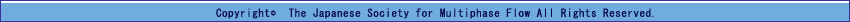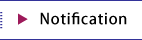Award Notice and Attention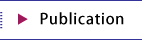Japanese Journal of Multiphase Flow Publication Contributions Rules of Contribution Making of Offset Manuscript Introduction lecture on multiphase flowExcutive Committee General Affairs Division Planning Division International Information Exchange Committee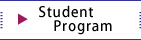Student Program Student Program News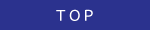TOP　＞　Publication　＞　Introduction lecture on multiphase flow > Dynamics of particle-fluid multiphase flows

# Dynamics of Particle-Fluid Multiphase Flows

-Basics of Numerical Simulation-

[ Japanese version ]

## Index

1. Classification (Lecture 1)
1. What is particle-fluid multiphase flow?
2. Classification based on particle-fluid interaction
3. Classification of modelling (continuum, discrete, DNS)
2. Particle-Fluid Interaction I (Transport Phenomena)
1. Differential operator, nabla, and Gauss divergence theorem (Lecture 2)
2. Momentum transfer (drag force, equation of particle motion) (Lecture 3)
3. Heat and mass transport (Nusselt number and Sherwood number) (Lecture 4)
3. Collision and Contact (Particle-Wall, Particle-Particle)
1. Hard (rigid) particle (particle-wall collision) (Lecture 5)
2. Hard (rigid) particle (particle-particle collision) (Lecture 6)
3. Elastic particle (Distinct Element Method : DEM) (Lecture 7)
4. Particle-Fluid Interaction II
1. Continuity equation and inertial term of Navier-Stokes equation (single phase) (Lecture 8)
2. Stress term of Navier-Stokes equation (single-phase) (Lecture 9)
3. Volume-averaged continuity equation (multi-phase) (Lecture 10)
4. Volume-averaged Navier-Stokes Equation (multi-phase) (Lecture 11)
5. Review of first law of thermodynamics and specific heat (Lecture 12)
6. Energy equation (Lecture 13)

## Lecture Videos

1. Classification (Lecture 1)
1. What is particle-fluid multiphase flow?
2. Classification based on particle-fluid interaction
3. Classification of modelling (continuum, discrete, DNS)
2. Particle-Fluid Interaction I (Transport Phenomena)
1. Differential operator, nabla, and Gauss divergence theorem (Lecture 2)
2. Momentum transfer (drag force, equation of particle motion) (Lecture 3)
3. Heat and mass transport (Nusselt number and Sherwood number) (Lecture 4)
3. Collision and Contact (Particle-Wall, Particle-Particle)
1. Hard (rigid) particle (particle-wall collision) (Lecture 5)
2. Hard (rigid) particle (particle-particle collision) (Lecture 6)
3. Elastic particle (Distinct Element Method : DEM) (Lecture 7)
4. Particle-Fluid Interaction II
1. Continuity equation and inertial term of Navier-Stokes equation (single phase) (Lecture 8)
2. Stress term of Navier-Stokes equation (single-phase) (Lecture 9)
3. Volume-averaged continuity equation (multi-phase) (Lecture 10)
4. Volume-averaged Navier-Stokes Equation (multi-phase) (Lecture 11)
5. Review of first law of thermodynamics and specific heat (Lecture 12)
6. Energy equation (Lecture 13)

▲page top